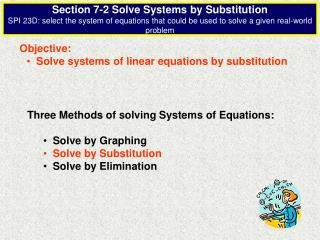Download PresentationObjective: Solve systems of linear equations by substitution

# Objective: Solve systems of linear equations by substitution - PowerPoint PPT PresentationDownload Presentation## Objective: Solve systems of linear equations by substitution

- - - - - - - - - - - - - - - - - - - - - - - - - - - E N D - - - - - - - - - - - - - - - - - - - - - - - - - - -
##### Presentation Transcript

1. Section 7-2 Solve Systems by SubstitutionSPI 23D: select the system of equations that could be used to solve a given real-world problem • Objective: • Solve systems of linear equations by substitution • Three Methods of solving Systems of Equations: • Solve by Graphing • Solve by Substitution • Solve by Elimination

2. Solve a System of Linear Equations by Substitution Substitute: Replace a variable with an equivalent expression containing the other variable. Solve the system of linear equations using substitution. y = - 4x + 8 y = x + 7 x + 7 1. Write an equation containing only one variable. y = = - 4x + 8 Substitute x + 7 for y. 2. Solve the equation for x. x = 0.2 3. Substitute the x value into either equation to find y. y = x + 7 y = 0.2 + 7 = 7.2

3. Solve a System of Linear Equations by Substitution Sometimes it is necessary to, first, solve one of the equations for a variable before using substitution. Solve the system of linear equations using substitution. 6y + 8x = 28 3 = 2x - y 1. Solve one of the equations for a variable. 3 = 2x – y 2x – 3 = y 2. Substitute the equation in step 1, into the remaining equation. 6y + 8x = 28 6 + 8x = 28 (2x – 3) 3. Solve for x. Substitute x into either equation to find y. x = 2.3 and y = 1.6

4. Real-world and Systems of Equations Suppose you are thinking about buying a car. Car A cost \$17,655 and you expect to pay an average of \$1230 per year for fuel and repairs. Car B costs \$15,900 and the average cost of fuel and repairs is \$1425 per year. After how many years are the total costs for the cars the same? 1. Write two equations to model the problem. C(y)= 1425y + 15,900 C(y)= 1230y + 17,655 2. Use substitution to solve. 1230y + 17,655 = 1425y + 15,900 17,655 - 15,900 = 1425y - 1230y 17,655 - 15,900 = 1425y - 1230y 1755 = 195y 9 = y The cost will be the same after 9 years.